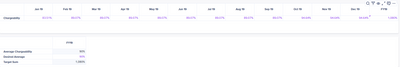# Ability to automatic change a specific value in a table based on changing a value on other tableedited December 2022 in Modeling

Hi everyone,

While building a model I came across a question.

I would like to know if it is possible to update a value of a table after filling another table. In this specific case I wanted that when I changed the "desired average" of the second table the Total Sum FY19 of the first table updated with the new value of the Total Sum that is in the second table. Basically, to change the value of the first table (FY19) automatically by the Total Sum of the second table when filling the desired average in the second table.Does anyone know if this is possible and how I could do it?

Thanks!

Tagged:

•edited December 2022

Hi Manuel,

The total year can not have a value without having the monthly input. Therefore, the "chargeability" line item of the first table should have a formula that takes the "desirable average" into account and splits it proportionally over the months.

If the formula option doesn't work, I'd add some conditional formatting to the first table to flag that there is a gap between the FY amount calculated and the FY total.

Hope that this would help.

Oumaima